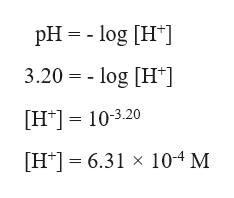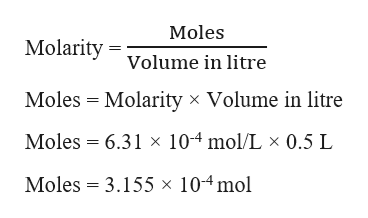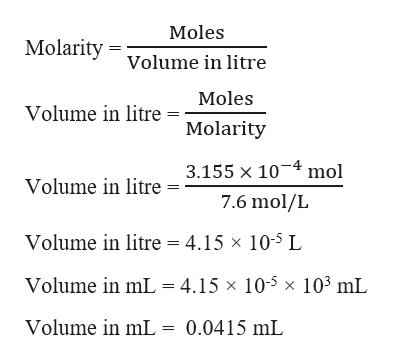# What volume (in mL) of 7.6 M HI would be required to make 500.0 mL of a solution with a pH of 3.20?

Question
1 views

What volume (in mL) of 7.6 M HI would be required to make 500.0 mL of a solution with a pH of 3.20?

check_circle

Step 1

Given,

Molarity of HI = 7.6 M = 7.6 mol/L

pH of the solution = 3.20

Volume of pH solution = 500.0 mL = 0.5 L  (1mL = 0.001 L)

From the pH, we can calculate the molarity of H+ ions in the solution as :help_outlineImage TranscriptionclosepH = - log [H*] 3.20 = - log [H*] [H*] = 10-3.20 %3D [H*] = 6.31 × 10-4 M fullscreen
Step 2

From the volume of the solution  given, we can calculate the moles of H+ ions :help_outlineImage TranscriptioncloseMoles Molarity = volume in litre Moles = Molarity × Volume in litre Moles = 6.31 x 104 mol/L × 0.5 L Moles = 3.155 × 104mol fullscreen
Step 3

HI is a strong acid and it will dissociate completely in solution to give H+ ion and I- ion.

HI (aq) → H+ (aq) + I- (aq)

[HI] = [H+] = [I-]

So, the number of moles of H+ ion in HI solution must be same as that of the H+ in the buffer solution to get a pH of...help_outlineImage TranscriptioncloseMoles Molarity = volume in litre Moles Volume in litre = Molarity 3.155 x 10-4 mol Volume in litre = 7.6 mol/L Volume in litre = 4.15 x 105 L Volume in mL = 4.15 × 10-5 × 10³ mL Volume in mL = 0.0415 mL fullscreen

### Want to see the full answer?

See Solution

#### Want to see this answer and more?

Solutions are written by subject experts who are available 24/7. Questions are typically answered within 1 hour.*

See Solution
*Response times may vary by subject and question.
Tagged in

### Equilibrium Concepts